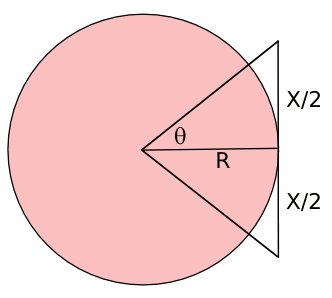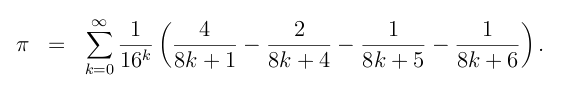Pi Day

March 14, 2012

Today is "Pi Day" (3.14). I wasn't among the many fans who likely stayed awake until 1:59 AM to get those last extra decimal digits, but I'm still a big fan of the mathematical constant, pi.

Pi appears to be an important part of the physical universe, since it appears in so many fundamental equations of physics. For example, the Einstein field equations of general relativity contain the constant (8πG/c4).

Pi must have weighed heavily on my mind in 2010, since I wrote three articles about it.
• Buffon's Needle, July 19, 2010
• Another Piece of Pi, July 28, 2010
• Pi is Forever, September 23, 2010
The last article somehow made it to the fourth page of 43,400 results on Google when I searched for hex digits of pi. It must be a niche area. More on hex digits of pi later in this article.

The first accurate estimates of pi were done by Archimedes, who used a geometrical approach of approximating a circle by inscribed and circumscribed polygons (see figure). You can do this for regular polygons of any number of sides, but Archimedes was limited to those with special angles, such as 30 degrees, for which he could calculate the tangent.

Today, using trigonometric tables, we can do this for any regular polygon. This doesn't make that much sense, since the trigonometric functions are calculated by series expansions, and so is pi.Principle behind the calculation of pi using a circumscribed polygon.

(Rendered by the author using Inkscape)

Using Archimedes' method, it's a simple matter to get the perimeters P of the polygons and ratio them to the radius R of the circle to get
π ≈ P/(2R),
Archimedes was able to calculate our elementary school value of pi, 22/7, using a circumscribed 96-sided polygon. Ptolomey did the same with a 360-sided polygon, getting the value, 3.141666..., that's correct to three decimal places.

Computer techniques have allowed a calculation of pi to 10,000,000,000,050 decimal digits. This was done by Shigeru Kondo, a Japanese systems engineer, on home-built hardware. This is truly overkill. Our present estimate of the diameter of the universe is 93 billion light-years (8.8 x 1026 meters), so we only need twenty-seven digits of pi to calculate the circumference of the universe to better than a meter, and thirty-eight digits to get a value of the circumference closer than the size of an atom.

In the past, if you wanted the n-th digit of pi, you needed to calculate all the digits leading up to n. This changed in 1997, when David Bailey, Peter Borwein and Simon Plouffe (BBP) published a formula that allows calculation of any digit of pi without calculation of all the preceding digits.[1-3]As the 16k term probably tipped you off, the equation gives pi in hexadecimal form.

I've posted C source code for calculation of the first 20,000 hex digits of pi in a previous article, but it's worth a few extra electrons to post the source code of an improved version. It's based on code published by David Bailey, and it will calculate these 20,000 hex digits in under a minute on most computers.

Mathematician, Clifford Pickover, published an interesting conjecture in May, 2003. This conjecture, which depends on the digits of pi being truly random, is as follows:
"Somewhere inside the digits of pi is a representation for all of us -- the atomic coordinates of all our atoms, our genetic code, all our thoughts, all our memories. Given this fact, all of us are alive, and hopefully happy, in pi. Pi makes us live forever. We all lead virtual lives in pi. We are immortal."
You can try to find parts of yourself in pi, at this web site.

References:

Linked Keywords: Pi Day; decimal digits; mathematical constant, pi; physical universe; fundamental equations; physics; Einstein field equations; general relativity; 2010; Buffon's Needle, July 19, 2010; Another Piece of Pi, July 28, 2010; Pi is Forever, September 23, 2010; Google; hex digit; Archimedes; geometry; polygon; regular polygon; tangent; trigonometric tables; series expansion; Inkscape; perimeter; radius; elementary school; Ptolomey; 10,000,000,000,050 decimal digits; Japanese; diameter of the universe; light-year; meter; size of an atom; David Bailey; Peter Borwein; Simon Plouffe; formula; C source code; electron; Clifford Pickover; conjecture; random.